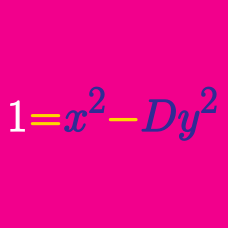Number Theory

# Quadratic Diophantine Equations: Level 4 Challenges

$$4$$ has the property that if one adds it to double its square, it yields a perfect square. In other words for natural numbers $$m,n$$:

$n^2 + n^2 + n = m^2$

There are four such $$n<10^6$$. If $$4$$ is the smallest $$n$$, what is the second smallest $$n$$?

What is the largest integer $$\displaystyle n$$ for which $$n^2+24n+16$$ is a perfect square?

$$365$$ can be written as a sum of $$2$$ consecutive perfect squares and also $$3$$ consecutive non-zero perfect squares: $365=14^2+13^2=12^2+11^2+10^2$

What is the next number with this property?

For how many positive integers $$n<10^6$$ is $$2\times n! \times (n+2)!$$ a perfect square?

Find the sum of all positive integers $$m$$ such that $$2^m$$ can be expressed as sums of four factorials (of positive integers).

Details and assumptions

The number $$n!$$, read as n factorial, is equal to the product of all positive integers less than or equal to $$n$$. For example, $$7! = 7 \times 6 \times 5 \times 4 \times 3 \times 2 \times 1$$.

The factorials do not have to be distinct. For example, $$2^4=16$$ counts, because it equals $$3!+3!+2!+2!$$.

×